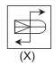>  Test: BITSAT Mock Test- 7

# Test: BITSAT Mock Test- 7

Test Description

## 150 Questions MCQ Test BITSAT Mock Tests Series & Past Year Papers | Test: BITSAT Mock Test- 7

 1 Crore+ students have signed up on EduRev. Have you?
Test: BITSAT Mock Test- 7 - Question 1

### Same current is flowing in two alternating circuits. The first circuit contains only inductance and the other contains only a capacitor. If the frequency of the e.m.f. of A.C. is increased, the effect on the value of the current will be

Test: BITSAT Mock Test- 7 - Question 2

### Ionization potential of hydrogen atom is 13.6 eV. Hydrogen atoms in the ground state are excited by monochromatic radiation of photon energy 12.1 eV. the spectral lines emitted by hydrogen atoms according to Bohr's theory will be

Detailed Solution for Test: BITSAT Mock Test- 7 - Question 2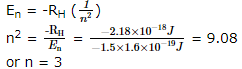Test: BITSAT Mock Test- 7 - Question 3

### Heavy water has molecular weight

Test: BITSAT Mock Test- 7 - Question 4
Two coils are placed close to eachother. The mutual inductance of the pair of coils depends upon
Test: BITSAT Mock Test- 7 - Question 5

An infinite number of identical capacitors, each of capacitance 1 μ F, are connected as in the figure. The equivalent capacitance between 'A' and 'B' is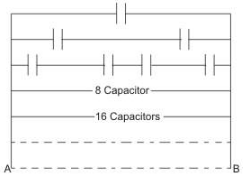Detailed Solution for Test: BITSAT Mock Test- 7 - Question 5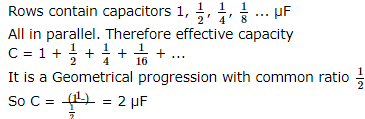Test: BITSAT Mock Test- 7 - Question 6
Consider the following statements concerning electrons :(I) Electrons are universal constitutents of matter (II) J.J. Thomson received the very first Nobel Prize in Physics for discovering the electron (III) The mass of the electron is about 1/2000 of that of a neutron (IV) According to Bohr the linear momentum of the electron is quantised in the hydrogen atom. Which of the above statements are NOT correct ?
Test: BITSAT Mock Test- 7 - Question 7
A cell of emf E has a resistance R connected across it, and a voltmeter measures the potential difference across the terminals of the cell is V. The internal resistance of the cell is
Test: BITSAT Mock Test- 7 - Question 8
If work function of metal is 3 eV then threshold wavelength will be
Detailed Solution for Test: BITSAT Mock Test- 7 - Question 8 Work function = hvoTherefore vo = WO/h = 3 x 1.602 x 10^-19 / 6.626 x 10^-34= 0.724 x 10^15/secThreshold Wavelength: λo =c/vo = 3x10^8 / 0.724 x 10^15 = 4.143 x 10^7 m
Test: BITSAT Mock Test- 7 - Question 9
Specific resistance of a wire depends upon
Test: BITSAT Mock Test- 7 - Question 10

If 4 * 1020 eV of energy is required to move a charge of 0•25 coluomb between two points, the p.d between them is

Test: BITSAT Mock Test- 7 - Question 11

On a rough horizontal surface, a body of mass 2 kg is given a velocity of 10 m/s. If the coefficient of friction is 0.2 and g=10 m/s2, the body will stop after covering a distance of

Detailed Solution for Test: BITSAT Mock Test- 7 - Question 11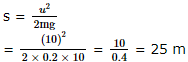Test: BITSAT Mock Test- 7 - Question 12
If acceleration due to gravity on the surface of a planet is two times that on surface of earth and its radius is double that of earth. Then escape velocity from the surface of that planet in comparison to earth will be
Test: BITSAT Mock Test- 7 - Question 13
If 10% of the current passes through a moving coil galvanometer of resistance 99 ohm, then the shunt resistance will be
Test: BITSAT Mock Test- 7 - Question 14
The absolute temperature of a gas is determined by
Test: BITSAT Mock Test- 7 - Question 15
Curie temperature is the temperature above which
Test: BITSAT Mock Test- 7 - Question 16

The temperature of a body falls from 50oC to 40oC in 10 minutes. If the temperature of the surroundings is 20oC Then temperature of the body after another 10 minutes will be

Test: BITSAT Mock Test- 7 - Question 17

A river water is flowing at 0.5 m-s⁻1. The shearing stress between horizontal layers at a distance of 10 cm of the river water is (Viscosity of water = 0.01 poise)

Test: BITSAT Mock Test- 7 - Question 18

If an air-bubble of radius 0.4 mm at a depth h in water having surface tension of 72*10⁻3 N-m⁻1 and density 1000 kg-m⁻3 is in equilibrium then, the value of h is (Take g=10 m-s⁻2)

Test: BITSAT Mock Test- 7 - Question 19
Which of the following affets the elasticity of a substance?
Test: BITSAT Mock Test- 7 - Question 20
A rocket is fired upward from the earth's surface such that it creates an acceleration of 19.6 m/sec2. If after 5 sec its engine is switched off, the maximum height of the rocket from earth's surface would be
Test: BITSAT Mock Test- 7 - Question 21

A stone projected with a velocity u at an angle θ with the horizontal reaches maximum height H₁. When it is projected with velocity u at an angle (π/2 -θ) with the horizontal, it reaches maximum height H₂. The relation between the horizontal range R of the projectile, H₁ and H₂ is

Test: BITSAT Mock Test- 7 - Question 22

The horizontal range is four times the maximum height attained by a projectile. The angle of projection is

Test: BITSAT Mock Test- 7 - Question 23

An impulse applied to a moving object with the force at an angle of 120 o with respect to the velocity vector. The angle between impulse vector and change in momentum vector is

Test: BITSAT Mock Test- 7 - Question 24
The focal lengths of the objective and eye lenses of a telescope are 200 cm and 5 cm respectively. The maximum power of the telescope will be
Test: BITSAT Mock Test- 7 - Question 25
The length of a simple pendulum is increased by 1%. Its time period will
Test: BITSAT Mock Test- 7 - Question 26
A simple pendulum executing simple harmonic motion is falling freely along with the support. Then
Test: BITSAT Mock Test- 7 - Question 27
A body A of mass M while falling vertically downwards under gravity breaks into two parts, a part B of mass M/3 and body C of mass 2M/3. The centre of mass of the bodies B and C taken together
Detailed Solution for Test: BITSAT Mock Test- 7 - Question 27 No horizontal external force is acting
∴ acm = 0
since vcm = 0
∴ Δxcm = 0
Test: BITSAT Mock Test- 7 - Question 28

The moment of inertia of a thin uniform circular disc about one of the diameters is I. Its moment of inertia about an axis perpendicular to the circular surface and passing through the centre is

Test: BITSAT Mock Test- 7 - Question 29
Moment of inertia of a uniform horizontal solid cylinder of mass M about an axis passing through its edge and perpendicular to the axis of the cylinder when its length is 6 times its radius 'R' is
Test: BITSAT Mock Test- 7 - Question 30

A point object O is placed at 15 cm in front of a glass rod having spherical end of radius of curvature 30 cm. The image would be formed at

Detailed Solution for Test: BITSAT Mock Test- 7 - Question 30

Here, μ1 = 1, μ2 = 1.5, u = - 15 cm,
R = + 30 cm, v = ?
Refraction from rearer to denser medium,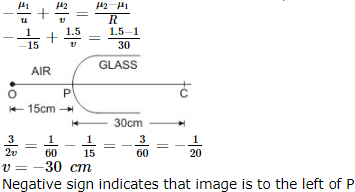Test: BITSAT Mock Test- 7 - Question 31

A fuse wire with radius 1 mm blows at 1.5 amp. The radius of the fuse wire of the same material to blow at 3A will be

Test: BITSAT Mock Test- 7 - Question 32
The internal energy of the gas increases when
Test: BITSAT Mock Test- 7 - Question 33
In which of the following systems of unit, Weber is the unit of magnetic flux.
Test: BITSAT Mock Test- 7 - Question 34

A reversible engine converts one-sixth of the heat into work. When temperature of sink is reduced by 62oC, the efficiency of the engine is doubled. The temperature of source and sink are

Test: BITSAT Mock Test- 7 - Question 35
When a source is linear, the wavefront is
Test: BITSAT Mock Test- 7 - Question 36

If the two slits in Young's experiment have width ratio 1:4, the ratio of intensity at maxima and minima in the interference pattern in

Detailed Solution for Test: BITSAT Mock Test- 7 - Question 36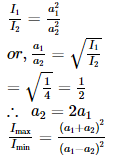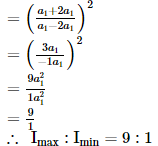Test: BITSAT Mock Test- 7 - Question 37
A standing wave having 3 nodes and 2 antinodes is formed between two atoms having a distance 1.21 Å between them. The wavelength of the standing wave is
Test: BITSAT Mock Test- 7 - Question 38
In a sinusoidal wave, the time required for a particular point to move from maximum displacement is 0.5 s. The frequency of wave is
Test: BITSAT Mock Test- 7 - Question 39
A stationary particle explodes into two particles of masses m₁ and m₂, which move in opposite directions, with velocities ν₁ and ν₂. The ratio of their kinetic energies (E₁/E₂) is
Test: BITSAT Mock Test- 7 - Question 40
A ball of mass 'm' moving with velocity 'V' collides with an identical ball at rest. After collision the first ball comes to rest. The speed of the other ball is
Test: BITSAT Mock Test- 7 - Question 41
Which of the following compounds does not react with metallic sodium?
Test: BITSAT Mock Test- 7 - Question 42
The organic compound which will answer the Fehling solution test is
Test: BITSAT Mock Test- 7 - Question 43

If r is the radius of first orbit, the radius of nth orbit of the H atom will be

Test: BITSAT Mock Test- 7 - Question 44

The no. of unpaired electrons in an element with electronic configuration 1s2,2s2,3p2 is

Test: BITSAT Mock Test- 7 - Question 45
Heterolytic fission of a covalent bond in organic molecules gives
Test: BITSAT Mock Test- 7 - Question 46
Point out the incorrect statement about resonance?
Test: BITSAT Mock Test- 7 - Question 47

Which of the following does not have sp2-hybridised carbon?

Test: BITSAT Mock Test- 7 - Question 48
Which of the following is an electrophile?
Test: BITSAT Mock Test- 7 - Question 49
The aldehyde which is formed during photosynthesis in plants is
Test: BITSAT Mock Test- 7 - Question 50
Test: BITSAT Mock Test- 7 - Question 51
In a reaction involving ring substitution of C6H5Y, the major product is meta isomer. The group Y can be
Detailed Solution for Test: BITSAT Mock Test- 7 - Question 51 Electron withdrawing groups favour meta substitution
- COOH group is metadirecting group
Test: BITSAT Mock Test- 7 - Question 52
For an exothermic reaction, the value of
Test: BITSAT Mock Test- 7 - Question 53
Which one of the following molecules is paramagnetic?
Test: BITSAT Mock Test- 7 - Question 54
when a catalyst is added to a reversible reaction in equilibrium state, the value of equilibrium constant
Test: BITSAT Mock Test- 7 - Question 55

1 dm3 of 2 M CH₃COOH is mixed with 1 dm3 of 3 M ethanol to form ester. The decrease in the initial rate if each solution is diluted with an equal volume of water would be

Test: BITSAT Mock Test- 7 - Question 56

Which of the following has highest first ionization potential?

Detailed Solution for Test: BITSAT Mock Test- 7 - Question 56

Nitrogen has highest first ionization potential. This is because N contains exactly half-filled p-orbitals which is extra stable. hence its first ionisation potential is high.
Electronic configuration of N: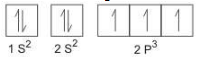Test: BITSAT Mock Test- 7 - Question 57

The densities of graphite and diamond at 298 K are 2.25 and 3.31 g-cm-3 respectively. If the standard free energy difference (ΔGo)is equal to 1895 J-mol-1, then pressure at which graphite will be transformed into diamond at 298 K is

Test: BITSAT Mock Test- 7 - Question 58
The chloramphenicol is an example of
Test: BITSAT Mock Test- 7 - Question 59
Which of the following is an inner orbital complex as well as diamagnetic in behaviour?
Detailed Solution for Test: BITSAT Mock Test- 7 - Question 59 Co3+ has 3d6 configuration and in the formation of the complex it assumes d2sp3 hybrid state and is, therefore, inner orbital complex
Test: BITSAT Mock Test- 7 - Question 60

The aqueous solution containing which of the following ions will be colourless?

Test: BITSAT Mock Test- 7 - Question 61
Which of the following is a synthetic element?
Test: BITSAT Mock Test- 7 - Question 62
The standard reduction potentials of 4 elements are given below.Which of the following will be the most suitable reducing agent?
I = -3.04 V, II = -1.90 V, III =0 V,IV = 1.90 V
Test: BITSAT Mock Test- 7 - Question 63
Of the five isomeric hexanes,the isomer which can give two monochlorinated compounds is
Test: BITSAT Mock Test- 7 - Question 64
A war gas Lewisite is formed by reaction of arsenic chloride with
Test: BITSAT Mock Test- 7 - Question 65

The chemical formula of lead sulphate is

Test: BITSAT Mock Test- 7 - Question 66
Silver nitrate solution is gradully added to an aqueous solution containing 0.01 M each of chloride ,bromide and iodide ions .The correct sequence in which the halides will be precipitated is
Test: BITSAT Mock Test- 7 - Question 67
Which of the following is an isomer of propanal?
Test: BITSAT Mock Test- 7 - Question 68
Ethyl bromide can be obtained by the action of HBr on
Test: BITSAT Mock Test- 7 - Question 69
The chemical used for cooling in refrigeration is
Test: BITSAT Mock Test- 7 - Question 70
Oxygen will directly react with each of the following elements except
Test: BITSAT Mock Test- 7 - Question 71
Liquid ammonia is used for refrigeration because
Test: BITSAT Mock Test- 7 - Question 72
Oxygen molecule is
Test: BITSAT Mock Test- 7 - Question 73
B(OH)₃ is
Test: BITSAT Mock Test- 7 - Question 74
Which is a protein?
Test: BITSAT Mock Test- 7 - Question 75
Which of the following oxides is paramagnetic?
Test: BITSAT Mock Test- 7 - Question 76
What are the metal ions present in carnalite?
Test: BITSAT Mock Test- 7 - Question 77
The vapour pressure of two liquids P ans Q are 80 and 60 torr, respectively. The total vapour pressure of solution obtained by mixing 3 moles of P and 2 moles of Q would be
Test: BITSAT Mock Test- 7 - Question 78
Select one correct statement. In the gas equation, PV = nRT
Test: BITSAT Mock Test- 7 - Question 79

Atomic weight of bromine is 80,0.1 mole of ethylene absorbs following gram of bromine

Detailed Solution for Test: BITSAT Mock Test- 7 - Question 79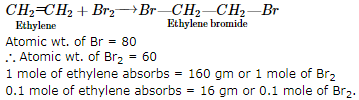Test: BITSAT Mock Test- 7 - Question 80
Which of the following collodial solution used in photography?
Test: BITSAT Mock Test- 7 - Question 81

The area bounded by the x-axis and the curve y = 4x - x2 - 3, is

Test: BITSAT Mock Test- 7 - Question 82
The term independent of x in the expansion of [(x1/3/2)+(x-1/5)]8 is
Test: BITSAT Mock Test- 7 - Question 83

The coordinates of a point from which tangents drawn to three circles x2+y2=1, x2+y2+8x+15=0, x2+y2+10y+24=0 are equal in length, are

Test: BITSAT Mock Test- 7 - Question 84

The number of common tangents to the circles x2 + y2 = 4 and x2 + y2 - 8x + 12 = 0 is

Test: BITSAT Mock Test- 7 - Question 85

The value of arg [(1-i√3)/(1+i√3)] is

Test: BITSAT Mock Test- 7 - Question 86

The differential equation of the family of curves y2=4a (x+a) is

Test: BITSAT Mock Test- 7 - Question 87
The general solution of the differential equation dy/dx=y/x is
Test: BITSAT Mock Test- 7 - Question 88
The number of points at which the function f(x)=|x-0.5|+|x-1|+tanx is not differentiable in (0,2) is
Test: BITSAT Mock Test- 7 - Question 89

y = Aex + Be2x + Ce3 x

satisfies the diferential equation

Test: BITSAT Mock Test- 7 - Question 90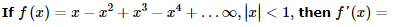Test: BITSAT Mock Test- 7 - Question 91

2/1! + 4/3! + 6/5! + .........∞ is equal to

Test: BITSAT Mock Test- 7 - Question 92
The area of the region bounded by the curves y = |x-1| and y = 3-|x| is
Test: BITSAT Mock Test- 7 - Question 93

The product of the perpendicular, drawn from any point on a hyperbola to its asymptotes is

Test: BITSAT Mock Test- 7 - Question 94
If f(x) = 0, when x is rational
x, when x is irrational,
g(x) = x, when x is rational
0, when x is irrational
then f - g is
Test: BITSAT Mock Test- 7 - Question 95

A house subtends a right angle on the window of its opposite house and the angle of elevation of the window from the foot of the former houses is 60o. If the distance between houses is 6 metres, then the height of the former house is

Test: BITSAT Mock Test- 7 - Question 96

If sin⁻1x+sin⁻1y=(2π/3), then cos⁻1x+cos⁻1y=

Test: BITSAT Mock Test- 7 - Question 97

The slope of the tangent at (x, y) to a curve passing through (2, 1) is (x2 + y2)/2xy, then the equation of the curve is

Test: BITSAT Mock Test- 7 - Question 98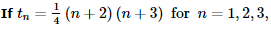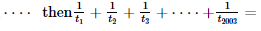Test: BITSAT Mock Test- 7 - Question 99
If A is a square matrix, then A - A is
Test: BITSAT Mock Test- 7 - Question 100

If each element of a 3 * 3 matrix is multiplie by 3, then the determinant of the newly formed matrix is

Test: BITSAT Mock Test- 7 - Question 101
If x + y = 12, then the minimum value of x2 + y2 is
Test: BITSAT Mock Test- 7 - Question 102
Which of the following is correct
Test: BITSAT Mock Test- 7 - Question 103

The angle between pair of lines represented by x2+2xy-y2=0 is

Test: BITSAT Mock Test- 7 - Question 104
If in a Binomial Distribution n = 4, P(X = 0) = 16/81, then P(X = 4)
Test: BITSAT Mock Test- 7 - Question 105

Two finite sets have m and n elements, the total number of subsets of the first set is 56 more than the total number of subsets of the second. The value of m and n are respectively

Detailed Solution for Test: BITSAT Mock Test- 7 - Question 105

Let A denote the first set and B denote the second set
We have, n(A) = 2m and n(B) = 2n
As per the question, we have
n(A) = 56 + n(B)
⇒ n(A) - n(B) = 56
⇒ 2m - 2n = 56
⇒ 2n (2m - n - 1)
⇒ 2n (2m - n - 1) = 8 * 7
⇒ 2n = 8 = 23 or (2m - n - 1) = 7
⇒ n = 3 or 2m - n = 8 = 23 = 26 - 3
⇒ n = 3 or m - n = 3
⇒ n = 3 or m = 6
Hence, the required values of m and n are 6 and 3 respectively

Test: BITSAT Mock Test- 7 - Question 106
The equation of the normal to the curve x2 = 4y at (1, 2) is
Test: BITSAT Mock Test- 7 - Question 107
P,Q,R and S have to deliver lecture, then in how many ways can the lectures be arranged?
Detailed Solution for Test: BITSAT Mock Test- 7 - Question 107 Total no. of ways delivering lectures = 4P4 = 4! = 24
Test: BITSAT Mock Test- 7 - Question 108

The parabola (y + 1)2 = a x − 2 passes through the point 1, − 2 . The equation of its directrix is

Test: BITSAT Mock Test- 7 - Question 109
A speaks truth in 75% cases and B speaks truth in 80% cases. The probability that they contradict each other in a statement is
Test: BITSAT Mock Test- 7 - Question 110

In Δ A B C , if the line joining the circumcentre 'O' and the incentre I is parallel to BC ,then cosB + cosC =

Test: BITSAT Mock Test- 7 - Question 111
If the roots of the equation 1/ x + p + 1/ x + q = 1/r are equal in magnitude but opposite in sign, then (p + q) equals
Test: BITSAT Mock Test- 7 - Question 112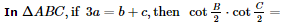Test: BITSAT Mock Test- 7 - Question 113
The sum of first 24 terms of an A.P. a1, a2, a3, .... ; if it is known that a1 + a5 + a10 + a15 + a20 + a24 = 225, is equal to
Test: BITSAT Mock Test- 7 - Question 114

If x, y, z are positive real numbers, then (x3/z) < (x3 + y3 + z3)/(x + y + z) < (z3/x) if

Test: BITSAT Mock Test- 7 - Question 115

The 6th term of a G.P. is 32 and its 8th term is 128 ; then the common ratio of the G.P. is

Detailed Solution for Test: BITSAT Mock Test- 7 - Question 115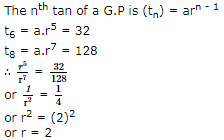Test: BITSAT Mock Test- 7 - Question 116
The foot of perpendicular from (2,4) on the line x+y=1 is
Test: BITSAT Mock Test- 7 - Question 117
If f (x) = log ((1 + x)/(1 - x)), then f (x) is
Test: BITSAT Mock Test- 7 - Question 118

The two curves x = y2, xy = a3 cut arthogonally at a point, then a2 =

Test: BITSAT Mock Test- 7 - Question 119
The distance between the parallel planes x + 2y - 3z = 2 and 2x + 4y - 6z + 7 = 0 is
Test: BITSAT Mock Test- 7 - Question 120
The shortest distance between the lines (x - 1)/2 = (y - 2)/3 = (z - 3)/4 and (x - 2)/3 = (y - 4)/4 = (z - 5)/5 is
Test: BITSAT Mock Test- 7 - Question 121
The period of sinθ-√3 cosθ is
Test: BITSAT Mock Test- 7 - Question 122
If vectors (i+j+k),(5i+3j-3k) and (2i+5j+9k) are the position vectors of vertices of any triangle then its perimeter is
Test: BITSAT Mock Test- 7 - Question 123
Find the Antonym of the word occurring in the sentence in capital letter as per the context.
The criminal was known to the police by VARIOUS names.
Detailed Solution for Test: BITSAT Mock Test- 7 - Question 123 'Various' means several and different
Test: BITSAT Mock Test- 7 - Question 124
The sine of the angle between the vectors 3i+2j-k and 12i+5j-5k is
Test: BITSAT Mock Test- 7 - Question 125

The value of cos 12o+cos 84o+cos 156o+cos 132o is

Test: BITSAT Mock Test- 7 - Question 126

All the values of x satisfying sin 2 x + sin 4 x = 2 sin 3 x are

Test: BITSAT Mock Test- 7 - Question 127
Find the Antonym of RISIBLE
Detailed Solution for Test: BITSAT Mock Test- 7 - Question 127 Meaning of the word ‘Risible is fit to be laughed at. Hence ‘Serious’ is the most suitable antonym of the given word. However, grotesque means comically or repulsively ugly or distorted.
Test: BITSAT Mock Test- 7 - Question 128
Fill in the blank with appropriate word.
Freedom and equality are the.....rights of every human being.
Test: BITSAT Mock Test- 7 - Question 129
Choose the one which can be substituted for the given words/sentences
The raison d' etre of a controversy is
Test: BITSAT Mock Test- 7 - Question 130
Choose the one which can be substituted for the given words/sentences
Study of birds
Test: BITSAT Mock Test- 7 - Question 131
Fill in the blank with appropriate word.
This is a.........translation of the speech.
Test: BITSAT Mock Test- 7 - Question 132
Spot the error.
Detailed Solution for Test: BITSAT Mock Test- 7 - Question 132 correct form is leading the blind instead of leading the blinds
Test: BITSAT Mock Test- 7 - Question 133
Spot the error.
Detailed Solution for Test: BITSAT Mock Test- 7 - Question 133 The correct statement would be 'besides your profession'
Test: BITSAT Mock Test- 7 - Question 134
Find the Synonym of the word occurring in the sentence in capital letter as per the context.
Valiant Vicky used to BOAST of his bravery to his beloved wife.
Detailed Solution for Test: BITSAT Mock Test- 7 - Question 134 Meaning of BOAST
To speak of (one's own achievements, possessions, etc) arrogantly adding things that may be false
Brag is also similar in meaning it is also speaking boastfully
Test: BITSAT Mock Test- 7 - Question 135
Find the Synonym of the word occurring in the sentence in capital letter as per the context.
His ALLEGIANCE to the party was suspected from the very beginning.
Detailed Solution for Test: BITSAT Mock Test- 7 - Question 135 'Allegiance' means continued support for somebody
Test: BITSAT Mock Test- 7 - Question 136
Spot the error.
Detailed Solution for Test: BITSAT Mock Test- 7 - Question 136 The correct statement is 'oil has been'
Test: BITSAT Mock Test- 7 - Question 137
Choose the word that does NOT match with the two given words.
Querulous : Peevish
Test: BITSAT Mock Test- 7 - Question 138
Complete the analogous pair.
Men : Messers :: ? : Women
Test: BITSAT Mock Test- 7 - Question 139

Group the following figures into three classes on the basis of identical properties.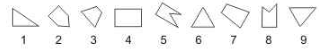Detailed Solution for Test: BITSAT Mock Test- 7 - Question 139

1,6,9, are all triangles.
3,4,7, are all four-sided figures.
2,5,8 are all five-sided figures.

Test: BITSAT Mock Test- 7 - Question 140
In each question, three sequences of letters/numerals are given which correspond to each other. Find out the letters/numerals that come in vacant places.
C _ B _ D _ A _ B B D D
2 _ _ 4 _ 3 4 _ ? ? ? ?
_ a _ c b a _ d _ _ _ _
Test: BITSAT Mock Test- 7 - Question 141
Find out the term in the number series which is wrong.
5, 27, 61, 122, 213, 340, 509
Detailed Solution for Test: BITSAT Mock Test- 7 - Question 141 The terms of the series are (23 - 3), (33 - 3), (43 - 3), (53 - 3), (63 - 3), (73 - 3), (83 -3).
So, 27 is wrong and must be replaced (33 - 3) i.e. 24
Test: BITSAT Mock Test- 7 - Question 142

Find the missing character.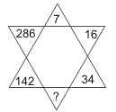Detailed Solution for Test: BITSAT Mock Test- 7 - Question 142

Clearly, we have : 7 * 2 + 2 = 16 ; 16 * 2 + 2 = 34 and so on.
So, missing nimber = 34 * 2 + 2 = 70.

Test: BITSAT Mock Test- 7 - Question 143

The strength of the electronics industry in Japan is the Japanese ability to organise production and marketing rather than their achievements in original research. The British are generally recognised as a far more inventive collection of individuals, but never seem able to exploit what they invent. There are many examples, from the TSR Z hovercraft, high speed train and Sinclair scooter to the Triumph, BSA and Norton motorcycle which all prove this sad rule. The Japanese were able, to 'exploit their strengths in marketing and development many years ago, and, their success was at first either not understood in the West or was dismissed as something which could have been produced only at their low price. They were sold because they were cheap copies of other peoples’ ideas churned out of a workhouse which was dedicated to hard grind above all else.

Q.It is evident from this passage that the strength of a country’s industry depends upon

Test: BITSAT Mock Test- 7 - Question 144

The strength of the electronics industry in Japan is the Japanese ability to organise production and marketing rather than their achievements in original research. The British are generally recognised as a far more inventive collection of individuals, but never seem able to exploit what they invent. There are many examples, from the TSR Z hovercraft, high speed train and Sinclair scooter to the Triumph, BSA and Norton motorcycle which all prove this sad rule. The Japanese were able, to 'exploit their strengths in marketing and development many years ago, and, their success was at first either not understood in the West or was dismissed as something which could have been produced only at their low price. They were sold because they were cheap copies of other peoples’ ideas churned out of a workhouse which was dedicated to hard grind above all else.

Q. The TSR Z hovercraft, high speed train, Sinclair Scooter, etc are the symbols of

Test: BITSAT Mock Test- 7 - Question 145

The strength of the electronics industry in Japan is the Japanese ability to organise production and marketing rather than their achievements in original research. The British are generally recognised as a far more inventive collection of individuals, but never seem able to exploit what they invent. There are many examples, from the TSR Z hovercraft, high speed train and Sinclair scooter to the Triumph, BSA and Norton motorcycle which all prove this sad rule. The Japanese were able, to 'exploit their strengths in marketing and development many years ago, and, their success was at first either not understood in the West or was dismissed as something which could have been produced only at their low price. They were sold because they were cheap copies of other peoples’ ideas churned out of a workhouse which was dedicated to hard grind above all else.

Q. According to the passage, prosperity in industry depends upon

Test: BITSAT Mock Test- 7 - Question 146

The strength of the electronics industry in Japan is the Japanese ability to organise production and marketing rather than their achievements in original research. The British are generally recognised as a far more inventive collection of individuals, but never seem able to exploit what they invent. There are many examples, from the TSR Z hovercraft, high speed train and Sinclair scooter to the Triumph, BSA and Norton motorcycle which all prove this sad rule. The Japanese were able, to 'exploit their strengths in marketing and development many years ago, and, their success was at first either not understood in the West or was dismissed as something which could have been produced only at their low price. They were sold because they were cheap copies of other peoples’ ideas churned out of a workhouse which was dedicated to hard grind above all else.

Q. The main theme of this passage is

Test: BITSAT Mock Test- 7 - Question 147

Select the correct mirror-image of the Figure (X) from amongst the given alternatives.

Q.